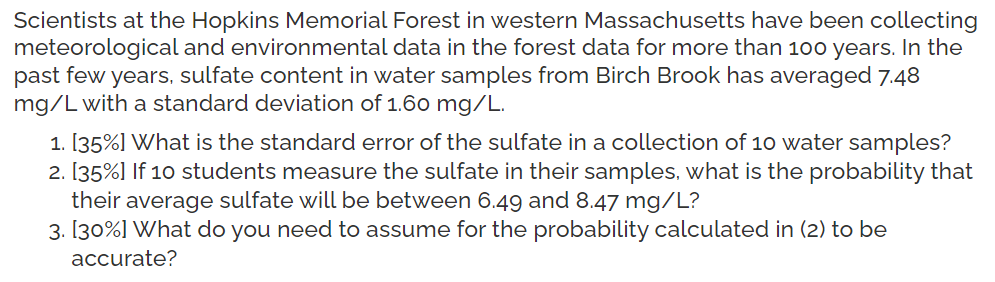# Question Solved1 AnswerScientists at the Hopkins Memorial Forest in western Massachusetts have been collecting meteorological and environmental data in the forest data for more than 100 years. In the past few years, sulfate content in water samples from Birch Brook has averaged 7.48 mg/L with a standard deviation of 1.60 mg/L. 1. [35%] What is the standard error of the sulfate in a collection of 10 water samples? 2. [35%] If 10 students measure the sulfate in their samples, what is the probability that their average sulfate will be between 6.49 and 8.47 mg/L? 3.130%] What do you need to assume for the probability calculated in (2) to be accurate?7KAVRN The Asker · Probability and StatisticsTranscribed Image Text: Scientists at the Hopkins Memorial Forest in western Massachusetts have been collecting meteorological and environmental data in the forest data for more than 100 years. In the past few years, sulfate content in water samples from Birch Brook has averaged 7.48 mg/L with a standard deviation of 1.60 mg/L. 1. [35%] What is the standard error of the sulfate in a collection of 10 water samples? 2. [35%] If 10 students measure the sulfate in their samples, what is the probability that their average sulfate will be between 6.49 and 8.47 mg/L? 3.130%] What do you need to assume for the probability calculated in (2) to be accurate?
More
Transcribed Image Text: Scientists at the Hopkins Memorial Forest in western Massachusetts have been collecting meteorological and environmental data in the forest data for more than 100 years. In the past few years, sulfate content in water samples from Birch Brook has averaged 7.48 mg/L with a standard deviation of 1.60 mg/L. 1. [35%] What is the standard error of the sulfate in a collection of 10 water samples? 2. [35%] If 10 students measure the sulfate in their samples, what is the probability that their average sulfate will be between 6.49 and 8.47 mg/L? 3.130%] What do you need to assume for the probability calculated in (2) to be accurate?